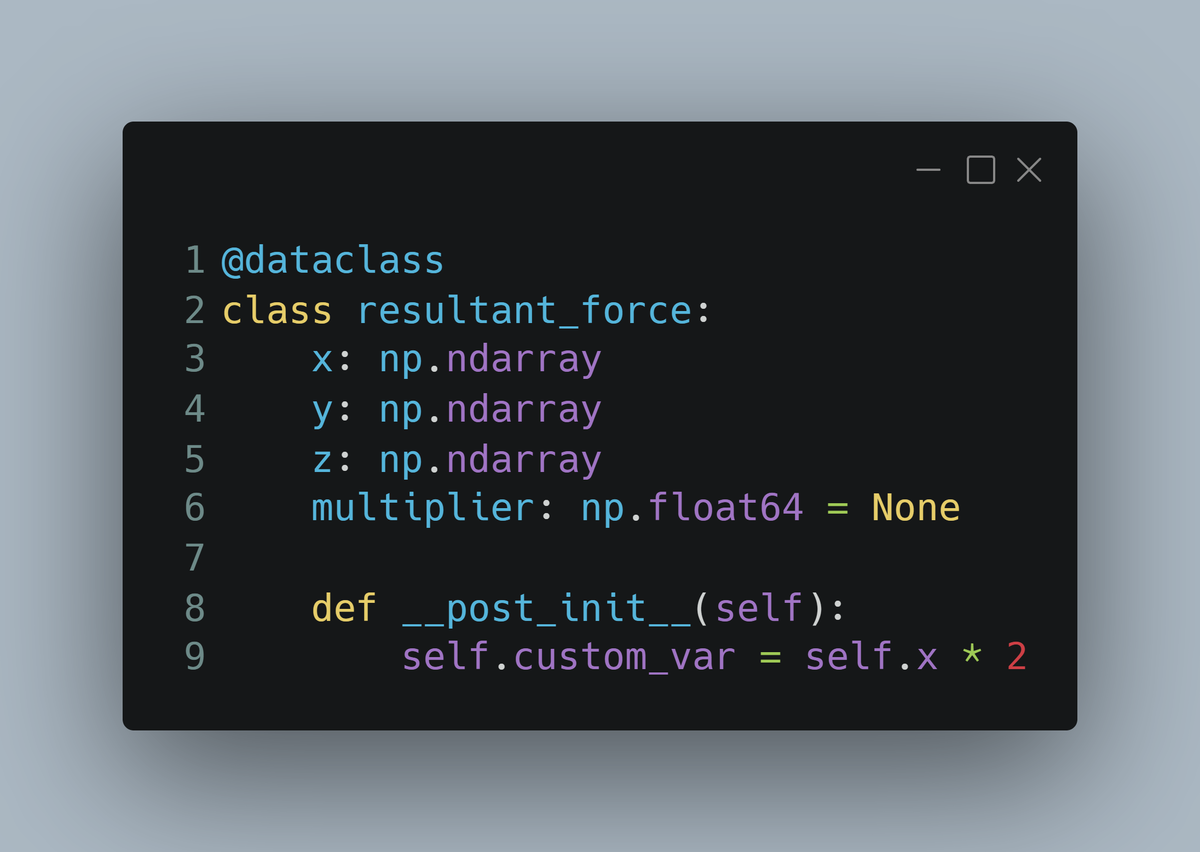# Are you using Data Classes?## Introduction

I don’t know about you but I have a tendency to store results in a dictionary and pass that around to functions when I need to. I have typically avoided creating classes for storing data as it always seemed a bit of overkill for the job at hand. Lots of repetitive code with little actual reward.

Here’s a rather simple example of what I mean, where I’m gathering all the results of interest into a single return item for a function. I find this easier than having multiple returns from multiple functions.


import numpy as np
from dataclasses import dataclass

def some_complex_function(forces, scale):

mult = np.pi**scale
complex_calculated_forces = forces * mult

result = dict()
result["val_x"] = complex_calculated_forces[:, 0]
result["val_y"] = complex_calculated_forces[:, 1]
result["val_z"] = complex_calculated_forces[:, 2]
result["mult"] = mult

return result

forces = np.random.random([1000,3])

calculated_forces = some_complex_function(forces, 4)

calculated_forces.keys()



This isn’t beautiful code, but it returns a single dict with all the related properties together, keeping the variable workspace a bit clearer in the process. Much handier if you need to pass this into several other functions later on in your workflow.

However, it’s not particularly re-usable and not great for modifying in future. Maybe a class would be a better option? But there’s so much effort involved in created a class I hear you say. All those __init__ and __repr__ methods that need to be defined, you may end up with a many lines of code for defining a very basic class.

## Data Classes

And that’s why data classes were introduced in python 3.7, to remove all that unnecessary boilerplate code required and just let you use the classes quickly.

So here’s my rather silly contrived example again, but this time using a fancy new data class.

import numpy as np
from dataclasses import dataclass

@dataclass
class resultant_force:
x: np.ndarray
y: np.ndarray
z: np.ndarray
multiplier: np.float64

def some_complex_function_using_dataclass(forces, scale):
mult = np.pi**scale
complex_calculated_forces = forces * mult

return resultant_force(*complex_calculated_forces.T, mult)

forces = np.random.random([1000,3])

force_dataclass = some_complex_function_using_dataclass(forces, 4)



I can now run dir(force_dataclass) on my result and see that it’s a fully fledged class :

['__annotations__',
'__class__',
'__dataclass_fields__',
'__dataclass_params__',
'__delattr__',
'__dict__',
'__dir__',
'__doc__',
'__eq__',
'__format__',
'__ge__',
'__getattribute__',
'__gt__',
'__hash__',
'__init__',
'__init_subclass__',
'__le__',
'__lt__',
'__module__',
'__ne__',
'__new__',
'__reduce__',
'__reduce_ex__',
'__repr__',
'__setattr__',
'__sizeof__',
'__slotnames__',
'__str__',
'__subclasshook__',
'__weakref__',
'multiplier',
'x',
'y',
'z']



There’s even a repe created for free! So I can quite easily query force_dataclass.multiplier and get Out: 97.40909103400242. What’s nice about this is now tht it’s a data class instead of a dictionary most IDE’s will autocomplete the dataclass fields for you, which is another bonus.

The other major benefit is now I have a nice reusable data container which I could make a little more generic and use in many places. I can do this because dataclasses also accept default values for fields. So I can change my previous class to something like this:

@dataclass
class resultant_force:
x: np.ndarray
y: np.ndarray
z: np.ndarray
multiplier: np.float64 = None

def some_complex_function_using_dataclass(forces, scale):
mult = np.pi**scale
complex_calculated_forces = forces * mult

return resultant_force(*complex_calculated_forces.T, mult)

def some_complex_function_using_generic_dataclass(forces):
complex_calculated_forces = forces * 2

return resultant_force(*complex_calculated_forces.T,)

force_dataclass = some_complex_function_using_dataclass(forces, 4)

generic_force_dataclass = some_complex_function_using_generic_dataclass(forces)



And now from one simple change I have a generic data structure that can be used in multiple places, passing in the additional variables when needed, otherwise they are set to None.

And data classes have one more nice trick where you can “embed” some calculation into the class.

@dataclass
class resultant_force:
x: np.ndarray
y: np.ndarray
z: np.ndarray
multiplier: np.float64 = None

def __post_init__(self):
self.custom_var = np.sum(self.x) / 3

def some_complex_function_using_generic_dataclass(forces):
complex_calculated_forces = forces * 2

return resultant_force(*complex_calculated_forces.T,)

force_dataclass = some_complex_function_using_dataclass(forces)



This now calculates whatever is in the __post_init__ method when the object is created. This is very handy if you always do some calculation with the data in the class, just simply embed the calculation within the class and the result will be there for you when you need it!

## Conclusion

These are just some very simple examples of how useful data classes can be for organising and improving your code. I love how the boilerplate of class creation is gone, and how they can make code more readable and easier to maintain.

There are many other features you would expect of a class and these are also included such as automatic __repr__ and object comparison. There’s also easy conversion to lists and dictionaries.

I suggest reading this post for a more detailed introduction to dataclasses and to see how they may help you.##### John P. Morrissey
###### Research Scientist in Granular Mechanics

My research interests include particulate mechanics, the Discrete Element Method (DEM) and other numerical simulation tools. I’m also interested in all things data and how to extract meaningful information from it.